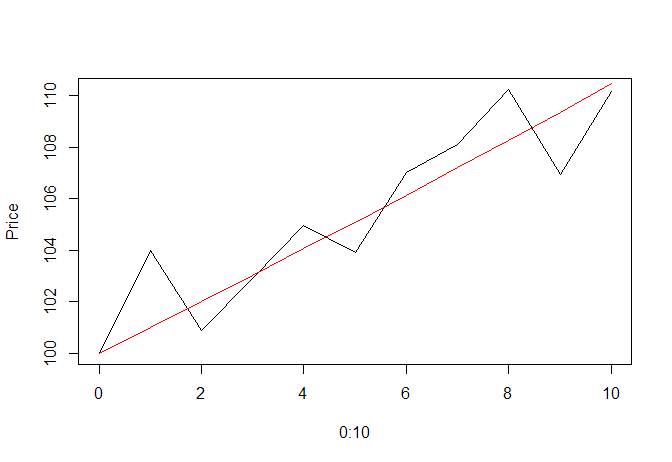Volatility is a very useful guide to many aspects of a stock's behaviour but I think for a lot of people it is either hard to understand, or maybe just seems to be a "magic" number which is difficult to interpret.

People sometimes express surprise that a stock has fallen by 40% and taken out a stop-loss when Stockopedia is reporting that the stock has a volatility of 160%. An understanding of volatility would greatly reduce any surprise in such a case.

Originally I planned to post some rules of thumb about what values of volatility mean, but I realised that simply perpetuates the idea that volatility is some deeply mysterious metric.

In fact it is based on a very simple - even obvious - idea which becomes much more user-friendly after you have tried working the volatility out for yourself.

So instead I will give what I hope is a very accessible explanation of how volatility is computed and then point out some rules of thumb afterwards.

## A Worked Example

Volatility is formally defined as the standard deviation of a stock's returns. So let's do a worked example.

A "return" is just the change in price divided by the original price, usually expressed as a percentage. So consider the following sequence of ten daily returns:

 1 2 3 4 5 6 7 8 9 10 returns 4 -3 2 2 -1 3 1 2 -3 3

This represents a stock increasing by 4% then falling by -3% and so on. Graphically the stock is doing this:To compute the volatility, we first find the 'mean' (i.e. average) daily change:

(4 -3 +2 +2 -1 +3 +1 +2 -3 +3)/10 = 1

On the chart above, I have plotted in red what the mean return sequence of prices would look like. As expected it gets to (nearly) the same end point but in a smooth way.

Then we subtract the mean from each return to get the deviation from the mean:

 1 2 3 4 5 6 7 8 9 10 returns 4 -3 2 2 -1 3 1 2 -3 3 deviations 3 -4 1 1 -2 2 0 1 -4 2

Now what we want is to find the mean (i.e. average) deviation from the mean.

But if we just add them up we will get zero:

(3 -4 +1 +1 -2 +2 +0 +1 -4 +2) = 0

(This should be obvious to you from the definition. We subtract the mean ten times so we have subtracted the sum of the returns from the sum of the returns. Hence zero.)

An obvious way…